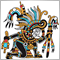# Using a Nested Cycle to Calculate Array Elements?448

This code example is taken straight from the MQL4 manual/tutorial.

```//--------------------------------------------------------------------
extern double Level=1.3200;                     // Preset level
string Text;                               // Array declaration
//--------------------------------------------------------------------
int init()                                      // Special funct. init()
{                                             // Assigning values
Text="one ";             Text="fifteen ";
Text="two ";             Text="sixteen ";
Text="three ";           Text="seventeen ";
Text="four ";            Text="eighteen ";
Text="five ";            Text="nineteen ";
Text="six ";             Text="twenty ";
Text="seven ";           Text="thirty ";
Text="eight ";           Text="forty ";
Text="nine ";            Text="fifty ";
Text="ten ";            Text="sixty";
Text="eleven ";         Text="seventy ";
Text="twelve ";         Text="eighty ";
Text="thirteen ";       Text="ninety";
Text="fourteen ";       Text= "hundred";
// Calculating values
for(int i=20; i<=90; i=i+10)                // Cycle for tens
{
for(int j=1; j<=9; j++)                  // Cycle for units
Text[i+j]=Text[i] + Text[j];          // Calculating value
}
return;                                     // Exit init()
}
//--------------------------------------------------------------------
int start()                                     // Special funct. start()
{
int Delta=NormalizeDouble((Bid-Level)/Point,0);// Excess
//--------------------------------------------------------------------
if (Delta<=0)                                // Price is not higher than level
{
return;                                   // Exit start()
}
//--------------------------------------------------------------------
if (Delta>100)                               // Price higher than 100
{
return;                                   // Exit start()
}
//--------------------------------------------------------------------
return;                                      // Exit start()
}
//--------------------------------------------------------------------```

The two 'for' cycles are used to calculate string values for the index 21-99 (excluding multiples of 10).

After the first iteration:

```i=20
j=1
Text[i+j]="twenty one"```

My understanding is that at this point, Text[i+j] stores the element "twenty one" as a result of the calculation 'i+j' on the first iteration.

But then on the second iteration, Text[i+j] will equal "twenty two".

So my question is essentially this:

After the first iteration, is the value of "twenty one" stored away as an element?

&

Will subsequent calculations of 'Text[i+j]' not impact this stored element? Or will a separate element effectively be stored to reflect the outcome of the new 'i+j' calculation?

&

If the above is true, what would be the index of this value?

---------------------------------------------------------------------------------------------------------------------------------------------------------------------------------------------------------------------------------------------------

Secondly:

In the final block, what specific steps are taken for 'Text[Delta]' to gain the string value of 'Delta'?

If Delta=72:

Does 'Delta' then refer back to the previous saved array element (as a result of the for cycles) for the string value of 72? If so, how specifically does this happen... I am close to understanding this in my mind but if someone could explain the specific process/steps then I would be very appreciative!

---------------------------------------------------------------------------------------------------------------------------------------------------------------------------------------------------------------------------------------------------

Thanks in advance to anyone who is kind enough to help out!34325

```//+------------------------------------------------------------------+
//| Script program start function                                    |
//+------------------------------------------------------------------+
void OnStart()
{
//---

for(int i=0;i<=100;i++)
{
Print("i=",i," ", NumToWords(i));
}

}
//+------------------------------------------------------------------+
string NumToWords(int num)
{
string kSpecialCases[] = {"ten", "eleven", "twelve", "thirteen", "fourteen", "fifteen", "sixteen", "seventeen", "eighteen", "nineteen"};
string kOnesPlaces[] = {"zero", "one", "two", "three", "four", "five", "six", "seven", "eight", "nine"};
string kTensPlaces[] = {"twenty", "thirty", "forty", "fifty", "sixty", "seventy", "eighty", "ninety"};

num=MathAbs(num);

if (10 <= num && num <= 19)
return kSpecialCases[num-10];

if (num < 10)
return kOnesPlaces[num];

else if (num<100)
{
if (num%10 == 0)
return kTensPlaces[num/10-2];
else
return kTensPlaces[num/10-2] + "-" + kOnesPlaces[num%10];
}

return "n/a";
}```34325

up to thousands

```string kSpecialCases[] = {"ten", "eleven", "twelve", "thirteen", "fourteen", "fifteen", "sixteen", "seventeen", "eighteen", "nineteen"};
string kOnesPlaces[] = {"zero", "one", "two", "three", "four", "five", "six", "seven", "eight", "nine", "ten"};
string kTensPlaces[] = {"twenty", "thirty", "forty", "fifty", "sixty", "seventy", "eighty", "ninety"};

//+------------------------------------------------------------------+
//| Script program start function                                    |
//+------------------------------------------------------------------+
void OnStart()
{
//---
for(int i=10995;i<=11021;i++)
{
Print("i=",i," ", NumToWords(i));
}
}
//+------------------------------------------------------------------+
string NumToWords(int num)
{
num=MathAbs(num);

if (num<100)
return LessHundred(num);

else if (num<1000)
return LessHundred(num/100) + " hundred "+((num%100>0)?LessHundred(num%100):"");

else if (num<100000)
return LessHundred(num/1000) + " thousand "+(((num%1000)/100>0)?LessHundred((num%1000)/100) + " hundred ":"")+((num%100>0)?LessHundred(num%100):"");

return "The number is greater than 99999";
}

//+------------------------------------------------------------------+
string LessHundred (int num)
{
if (10 <= num && num <= 19)
return kSpecialCases[num-10];

if (num < 10)
return kOnesPlaces[num];

else if (num<100)
{
if (num%10 == 0)
return kTensPlaces[num/10-2];
else
return kTensPlaces[num/10-2] + "-" + kOnesPlaces[num%10];
}
return"";
}```448

Taras Slobodyanik:

up to thousands

Thanks.34325

up to billions)

```string kSpecialCases[] = {"ten", "eleven", "twelve", "thirteen", "fourteen", "fifteen", "sixteen", "seventeen", "eighteen", "nineteen"};
string kOnesPlaces[] = {"zero", "one", "two", "three", "four", "five", "six", "seven", "eight", "nine", "ten"};
string kTensPlaces[] = {"twenty", "thirty", "forty", "fifty", "sixty", "seventy", "eighty", "ninety"};

//+------------------------------------------------------------------+
//| Script program start function                                    |
//+------------------------------------------------------------------+
void OnStart()
{
//---
for(long i=INT_MAX-50;i<=INT_MAX;i++)
{
Print("i=",i," ", NumToWords(i));
}
}
//+------------------------------------------------------------------+
string NumToWords(long num)
{
string txt="";
num=MathAbs(num);

if(num<1000000000000)
{
//billions
if(num>=1000000000)
{
long tmp=num/1000000000;
txt+=LessThousand((int)tmp)+" billion ";
num%=tmp*1000000000;
}

//millions
if(num>=1000000)
{
long tmp=num/1000000;
txt+=LessThousand((int)tmp)+" million ";
num%=tmp*1000000;
}

//thousands
if(num>=1000)
{
long tmp=num/1000;
txt+=LessThousand((int)tmp)+" thousand ";
num%=tmp*1000;
}

//hundreds
if(txt=="" || num>0)
txt+=LessThousand((int)num);
}

else txt="The number is greater than trillion.";

return (txt);
}

//+------------------------------------------------------------------+
string LessThousand (int num)
{
string txt="";

if (num>=100)
{
int tmp=num/100;
while(tmp>=10)
tmp/=10;

txt=kOnesPlaces[tmp]+" hundred ";
num%=(tmp*100);
}

if (10 <= num && num <= 19)
txt+=kSpecialCases[num-10];

else if (num < 10)
txt+=(num==0 && txt!="")?"":kOnesPlaces[num];

else
{
if (num%10 == 0)
txt+=kTensPlaces[num/10-2];
else
txt+=kTensPlaces[num/10-2] + "-" + kOnesPlaces[num%10];
}

return(StringTrimRight(txt));
}```